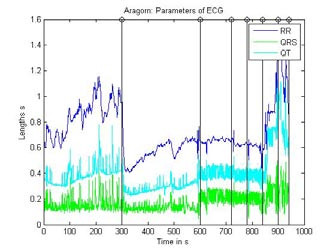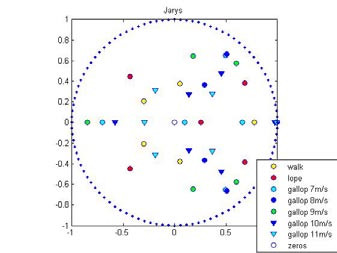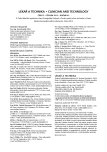# Linear Modelling of Cardiovascular Parameter Dynamics during Stress-Test in Horses

Authors: Terézia Hodásová 1,2;  Jiří Holčík 1,3
Authors‘ workplace: Institute of Biostatistics and Analyses, Masaryk University, Brno, Czech Republic 1;  Masaryk University, Brno, Czech Republic Department of Mathematics and Statistics of the Faculty of Science 2;  Institute of Measurement Science, Slovak Academy of Sciences, Bratislava, Slovakia 3
Published in: Lékař a technika - Clinician and Technology No. 2, 2012, 42, 15-18
Category: Conference YBERC 2012

## Overview

This paper deals with a processing of ECG signal parameters and modelling the parameter dynamics during the stresstest in horses. The standard Box-Jenkins methodology was applied for the linear model design. The order of the AR model (equal to 6) was estimated according to properties of autocorrelation and partial autocorrelation functions of the ECG parameter time series. Further, frequency responses of the model systems as well as distribution of their poles and zeros were determined for different stages of the stress test examination. The preliminary examination of the computed data and their dynamics indicates that they can carry useful information for evaluation the health and fitness conditions of the examined horses. All the computational procedures were implemented in MATLAB®.

Keywords:
Stress-test examination in horses, ECG signal parameters, stationary process, transfer function, AR model

## Introduction

The aim of the work was to find proper mathematical model of ECG parameter dynamics during the stresstest in horses. The stress-test examination consists of several stages. It starts with walk, lope and then gallop stages follow. The gallop stages ordinarily take 1 or 2 minutes starting with speed of 7m/s. After that the treadmill speed increases by 1 m/s usually up to 10 or 11 m/s.

The parameters of ECG signal change depending on the stress-test load. So the question is: is there any functional dependency based on the ECG signal parameters, which could describe the dynamics of the ECG parameters and which could characterise either the fitness level of the horse or state of its health?

Cardiovascular system condition can be characterised by several ECG parameters as the lengths of RR and/or QT intervals or duration of QRS complexes. In spite of the fact that all of these parameters were available, in this paper the lengths of RR intervals were considered only. It is because this parameter provides vets with a very fundamental information on the heart rate, its variability and ways of cardiovascular control.

The problematic of modelling dynamic ECG parameters is neglected part, therefore there were not known related works.

## Signal processing

### Data descriptions

The parameters were calculated from ECG signal recorded throughout stress-test . The determined irregularly sampled parameter time series were then resampled by frequency of 10 Hz for next processing (Fig.1). The origin samples were linear interpolated to obtain the continuous signal and this signal was in next step sampled by frequency of 10 Hz to obtain equidistant time series.Fig. 1 : Parameters of ECG signal recorded during the stress-test in horses separated by steps.

### Stationarity and frequency spectrum

The basic well known approach for a description of a time series dynamics is based on the Box-Jenkins methodology. However, the approach prefers if the data represent stationary processes. That is why it was necessary to test how severe the non-stationarity of stress test process is.

There are several types of tests for stationarity. Testing stationarity in the mean proved to be sufficient for our purpose because of data character. Stationarity in the mean means that the value of the data mean does not depend on time. Therefore the analysed time series were divided to four intervals of the same lengths. Values of the mean were calculated for each interval and compared. Then the ANOVA test was used and the hypotheses that the values are equal, was rejected with p-value p=0 on significance level 0.05. The fact signified non-stationarity of the process and that is why the data had to be preprocessed to eliminate a data drift component.

To model the drift the frequency approach was used based on knowledge of time series frequency spectrum (Fig. 2).

There are some significant frequency clusters in the depicted spectrum. Each of them describes specific process as breathing during the stress-test or noise generated by some technical artificial sources (electrode contact artefacts, movement of ECG leads, etc).

From the Fig. 2 it is obvious that the most significant cluster is that at very low frequencies, typical for the slow drift in the data. Shape of the frequency spectrum can be also used for specification of the cut-off frequency of a low-pass filter that can be applied to remove the drift. It was determined as a frequency, the absolute value of which had the biggest difference from the previous sample. Further, order of the moving average filter (that was planned to use for removing of the data drift) had to be chosen. Provided filter with Hamming window  and sampling frequency of 10 Hz the degree of 700 samples proved to be of the best performance based on the trial and error method. If the degree was lower, the higher frequencies were much more attenuated. The trend obtained by filtering signal using the low-pass filter with Hamming window is shown in Fig. 3.

It is possible to see corresponding changes in the frequency spectrum in the Fig. 4. It is obvious from the figure, that the higher frequencies were really not inconveniently influenced.Fig. 4: Frequency spectrum of signal with and without the drift.

The stationary signal after drift elimination (by subtracting estimated drift from the original RR interval time series) is shown in the Fig. 3, too.

The test of stationarity in mean for the signal without drift does not rejected the null hypothesis, the p-value was p=0.1928 on significance level 0.05. That meant, that the signal without drift could be supposed to represent a stationary process.

### Time series model

The proper linear model describing the RR interval data was looked for generally in the ARMA system family.

As it was mentioned before, the dynamics of parameters is connected to changes of the treadmill speed. Therefore the signal was divided into parts belonging to each step of the stress-test before next processing. These parts are obvious in the Fig. 3.

Each data interval was modelled individually, but the structure of the model was the same for all the cases. To determine such a structure it was necessary to decide which type of the model is the most applicable for the processed experimental data, then the order of model had to be set.

Both the tasks were solved by means of properties of an autocorrelation (ACF) and partial autocorrelation (PACF) function (see Fig. 5), .Fig. 5: ACF and PACF for step of gallop with speed 7 m/s.

For every stress-test step the estimated ACF was quickly decreasing curve shaped as attenuated sinusoid. Such a type of ACF function is typical for an autoregressive process, so the AR structure of the model proved to be the best choice. The degree of 6 of the AR model was estimated using the graph of PACF made for each part. It is not so clear from the Fig. 5. PACFs for all the stress-test steps were considered and rather simpler model than that with more complicated structure (higher order) was finally chosen.

On both of these graphs the 95% confidence intervals (blue lines) are shown. This interval is useful especially for estimation the degree of model. The values of lag numbers represent the model coefficients. If their values are outside of the confidence interval, then they are statistically significant and therefore they had to be included into the model.

### Characteristics of AR(6)

The AR model of the order of 6 is defined by the difference equation according to eq. (1), .

and its transfer function is

Character of the transfer function can be also described by a map of its zeros and poles (see Figs. 6, 7, 8). In Fig. 6 there are obvious some clusters of poles that belong to transfer functions for different stress-test stages. These groups describe the movement of one pole (sometimes the real pole splits into two complex conjugate poles and vice versa). So the clusters can be used to describe the trajectory of pole dynamics.Fig. 7: Map of zeros and poles of the transfer function for horse Line Honey.Fig. 8: Map of zeros and poles of the transfer function for horse Jarys.

The question is, if this map can be useful for obtain the fitness level in horses. Let us examine the maps for two other horses, (Fig. 7 and 8). The pole map structure is different in both the figures. The most concentrated poles in one place are in Fig.7 (Line Honey, well trained athlete with the best fitness level). That demonstrates the low dynamics of heart rate (RR intervals) in successive steps of the stress-test.

In the Fig. 8 (Jarys, horse with the worst level of fitness in the group of examined horses) the concentration of poles is rather poor, the poles are distributed within the whole unit circle. That describes high level dynamics of the parameter.

The concentration of poles for horse named Aragorn (Fig.6) is somewhere in a middle. Aragorn runs the races of the 3rd category. The previously mentioned examples demonstrate that the movement of poles of the model describing time series of RR intervals could provide us with useful information for assessing the fitness level in horses.

Position of pole clusters in the complex Z plane (for example defined by an angle between real axis and the line connecting origin of the coordinates and centre of the pole cluster) characterizes also frequencies of processes that influence the heart rate. As it can be seen in all the mentioned figures the examined horses could be also assessed by means of such a parameter.

## Conclusion

Experiments and calculations done with the experimental data up to now demonstrate that AR models and their characteristics could be considered as a useful tool for assessing especially fitness level in horses.

To verify such an idea it will be necessary to verify the hypothesis with larger set of experimental signals. Then the algorithms for machine classification of fitness level in horses will be developed.

### Acknowledgement

The research was partly granted by the project of the Czech Science Foundation No.102/09/H083: „Information Technology in Biomedical Engineering“ and by the VEGA project No. 2/0210/10 “Methods and Systems for Multichannel Measurement and Evaluation of Bioelectric Signals of Heart and Brain”.

Terézia Hodásová

Institute of Biostatistics and Analyses

Masaryk University

Kamenice 3, 62500 Brno

E-mail: 211192@mail.muni.cz

Tel. +420 775 119 908

##### Sources

 Svačinová J., Evaluation of Stress Test ECG Signal Parameters in Horses. [Diploma Thesis], Brno, Masaryk University, 2011. (in Czech)

 Box, G. E., Jenkins, G. M., Reinsel, G. C. : Time Series Analysis Forecasting and Control. 4th. ed., Hoboken, New Jersey: John Wiley & Sons, Inc. 2008. ISBN 978-0-470-27284-8.

 Forbelská, M.: Stochastic modelling of one-dimensional time series. 1st ISBN 978-80-210-4812-6. ed., Brno: Masaryk University, 2009. (in Czech)

 Bitschnau, C. et al.: Performance parameters and post excersice heart rate recovery in Warmblood sports horses of different performance level. Equine veterinary journal 42, 2010. 17-22

 Harris, F. J.: On the use of windows for harmonic analysis with the discrete Fourier transform. IEEE vol. 66, 1978. 51- 83

##### Labels
Biomedicine

Article was published in

### The Clinician and Technology Journal2012 Issue 2